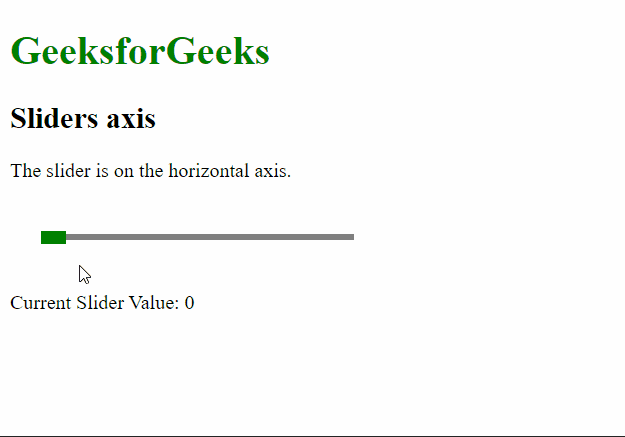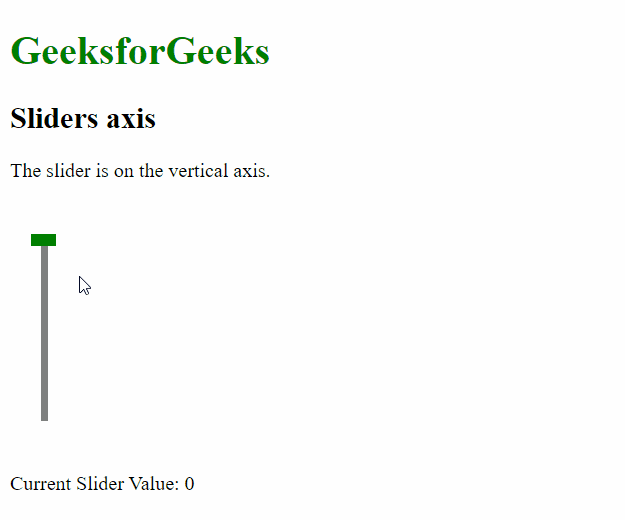# script.aculo.us Sliders Axis Option

• Last Updated : 20 Nov, 2020

The script.aculo.us library is a cross-browser library that aims to improving the user interface of a website. The Slider controls are thin tracks that allow the user to input values. It is done by defining a range of values that can be selected by the user by dragging the handle to the appropriate value.

The Sliders Axis option is used to define the axis or direction that the slider is supposed to move in. This option accepts a string value that can be set to ‘horizontal’ or ‘vertical’ depending on the direction required.

Hey geek! The constant emerging technologies in the world of web development always keeps the excitement for this subject through the roof. But before you tackle the big projects, we suggest you start by learning the basics. Kickstart your web development journey by learning JS concepts with our JavaScript Course. Now at it's lowest price ever!

Syntax:

```{ axis : horizontal | vertical }
```

Values: This option has two values as mentioned above and described below:

• horizontal: This would define the direction of the slider to be horizontal. It is the default value.
• vertical: This would define the direction of the slider to be vertical.

Example 1:

## HTML

 ```<``html``>`` ` `<``head``>``    ````    ``<``script` `type``=``"text/javascript"` `        ``src``=``"prototype.js"``>``    ```` ` `    ``<``script` `type``=``"text/javascript"` `src``=``"scriptaculous.js?load = slider"``>``    ```` ` `    ````    ``<``style` `type``=``"text/css"``>``        ``.track {``            ``width: 250px;``            ``background-color: gray;``            ``height: 5px;``            ``position: relative;``        ``}`` ` `        ``.track .handle {``            ``width: 20px;``            ``height: 10px;``            ``background-color: green;``            ``cursor: move;``            ``position: absolute;``            ``top: -2px;``        ``}`` ` `        ``.pad {``            ``padding: 25px;``        ``}``    `````` ` `<``body``>``    ``<``h1` `style``=``"color: green;"``>``        ``GeeksforGeeks``    ```` ` `    ``<``h2``>Sliders axis`` ` `    ``<``p``>``        ``The slider is on the ``        ``horizontal axis.``    ```` ` `    ``<``div` `class``=``"pad"``>``        ``<``div` `id``=``"track-hor"` `class``=``"track"``>``            ``<``div` `id``=``"handle-hor"` `class``=``"handle"``>``            ````        ````    ```` ` `    ``<``p``>Current Slider Value:``        ``<``span` `id``=``"out"``>0``    ```` ` `    ``<``script` `type``=``"text/javascript"``>``        ``new Control.Slider('handle-hor',``            ``'track-hor', {``            ``range: \$R(1, 100),`` ` `            ``// Set the axis of the slider``            ``// to be horizontal ``            ``axis: 'horizontal',`` ` `            ``onSlide: (val) => {``                ``document.querySelector("#out")``                    ``.textContent = val;``            ``}``        ``});``    `````` ` ``

Output:Example 2:

## HTML

 ```<``html``>`` ` `<``head``>``    ````    ``<``script` `type``=``"text/javascript"` `        ``src``=``"prototype.js"``>``    ```` ` `    ``<``script` `type``=``"text/javascript"` `src``=``"scriptaculous.js?load = slider"``>``    ```` ` `    ````    ``<``style` `type``=``"text/css"``>``        ``.track {``            ``width: 5px;``            ``background-color: gray;``            ``height: 150px;``            ``position: relative;``        ``}`` ` `        ``.track .handle {``            ``width: 20px;``            ``height: 10px;``            ``background-color: green;``            ``cursor: move;``            ``position: absolute;``            ``left: -8px;``        ``}`` ` `        ``.pad {``            ``padding: 25px;``        ``}``    `````` ` `<``body``>``    ``<``h1` `style``=``"color: green;"``>``        ``GeeksforGeeks``    ```` ` `    ``<``h2``>Sliders axis`` ` `    ``<``p``>The slider is on the vertical axis.`` ` `    ``<``div` `class``=``"pad"``>``        ``<``div` `id``=``"track-vert"` `class``=``"track"``>``            ``<``div` `id``=``"handle-vert"` `class``=``"handle"``>``            ````        ````    ```` ` `    ``<``p``>Current Slider Value:``        ``<``span` `id``=``"out"``>0``    ```` ` ` ` `    ``<``script` `type``=``"text/javascript"``>`` ` `        ``new Control.Slider('handle-vert',``            ``'track-vert', {``            ``range: \$R(1, 100),`` ` `            ``// Set the axis of the slider``            ``// to be vertical ``            ``axis: 'vertical',`` ` `            ``onSlide: (val) => {``                ``document.querySelector("#out")``                    ``.textContent = val;``            ``}``        ``});``    `````` ` ``

Output:My Personal Notes arrow_drop_up